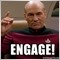# OnCalculate() Copying from the rates arrays2397

I need to copy the last ten highs from the high[] array in OnCalculate()

```   int Length = 10;
double ahigh[];
ArrayResize(ahigh,Length);
ArrayCopy(ahigh,high,0,0,Length);
for(int i=0; i<Length; i++)
{
Print("high[",i,"] = ",high[i]," ahigh[",i,"] = ",ahigh[i]);
}
int compare = ArrayCompare(high,ahigh,0,0,Length);
Print("ArrayCompare = ",compare);```

When I print them it looks ok but ArrayCompare() returns -1 why would that be ?

ArrayCompare = -1
high = 1.3748 ahigh = 1.3748
high = 1.3811 ahigh = 1.3811
high = 1.3861 ahigh = 1.3861
high = 1.3898 ahigh = 1.3898
high = 1.3905 ahigh = 1.3905
high = 1.3862 ahigh = 1.3862
high = 1.3833 ahigh = 1.3833
high = 1.385 ahigh = 1.385
high = 1.3864 ahigh = 1.3864
high = 1.3822 ahigh = 1.3822

Also if I call a value from the spread[] array,

`Print(spread);`

I get array out of range error in the experts log.187

(after 4 years now...呵呵)      i'm also troubled by this error...until i realized that the "spread[]" array may not be populized by MT4.

you can see this by:

`Print(ArraySize(spread));`20207

There is a difference between the arrays passed to OnCalculate (e.g. low[]) and the predefined variables (e.g. Low[].) The predefined variables are all ordered AsSeries. The passed arrays have no default direction.
To determine the indexing direction of time[], open[], high[], low[], close[], tick_volume[], volume[] and spread[], call ArrayGetAsSeries(). In order not to depend on default values, you should unconditionally call the ArraySetAsSeries() function for those arrays, which are expected to work with.
Event Handling Functions - Functions - Language Basics - MQL4 Reference1103

As I see it,

Bad decision to let those arrays passed to OnCalculate() be whatever

Those arrays should've have AsSeries = 1

It only brings unnecessary confusion.
Makes you wonder why the need for AsSeries in the first place,

but

...whatever